# Predicting future values with Excel Charts

Excel can help you make predictions about future values, or help you spot a linear trend. What we'll do in this section is set up something called a Trendline. We'll use an X, Y Scatter chart for this. We'll take a look at future income predictions based on what was earned in previous years. If you're a bit confused, don't worry: it will all become clear as we go along.

Type the following headings into cells A1 to C1:

Year
Years since 2006
Income

Format the cells, if you prefer. Your spreadsheet will then look like this: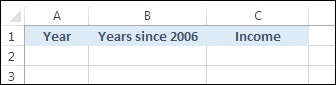Enter the years 2006 to 2019 into cells A2 to A15:As an X axis for our chart, we can have the years since 2006. These values will be used in a later formula. In Cells B2 to B15 enter the values 0 to 13:We now need some income values for the years 2006 to 2013. This is income that has actually been earned, rather than income that might be earned in the future. We'll then use this hard data to predict future values. Enter some income values, then, into cells C2 to C9. We made up the following values:We're now ready to insert an X, Y Scatter chart.

Highlight the cells B1 to C9:This will be the data for our chart.

From the top of Excel, click on the Insert ribbon. From the Charts panel, locate and click on the Scatter charter icon. The icon looks like this: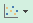Select the first item to get a chart with just dots:(If you can't see the icon above, click on Recommended Charts. Switch to the All Charts tab, then select X Y Scatter).

A new chart will then appear on your spreadsheet. It should look like this: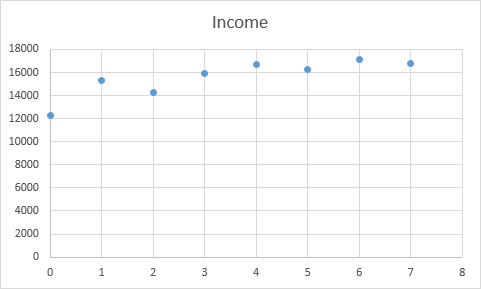The figures along the bottom, the X Axis, are our years since 2006. The figures on the Y Axis are our income values. The first dot, the one on the far left, tells us that we made just over 12000 at Year 0, (Year 0 is 2006). At Year 1 (2007) we made just under 16000. At Year 2 (2008) we made just over 14000, and so on.

All these dots seem to form a loose line going up from the left. You could add a line yourself using the Shapes item on the Illustrations panel. What you'll then have done is to create a linear regression.

Rather than add the line ourselves, however, Excel can add the line for us. Not only that, it can give us the formula it used to create the line. We can use that formula to predict future incomes.

Click on your chart to highlight it. You should see three icons appear on the right, in Excel 2013 and 2016. (See below for Excel 2007 and Excel 2010.) Click on the Plus symbol, and put a check in the box for Trendline: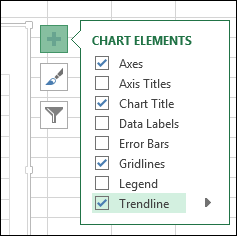When you check Trendline, you should see a line appear on your chart:To get the line in Excel 2007 and 2010, select your chart then click on the Layout tab. From the Analysis panel, click the Trendline option. From the Trendline menu, select Linear Trendline.

The line represents Excel's best fit for a linear regression. It's trying to put as many as the dots as it can as close to the line as possible.

To see the equation Excel used, click on the Plus symbol again (Excel 2013 and Excel 2016). Then click on the arrow to the right of Trendline. A new menu appears. Select More Options at the bottom: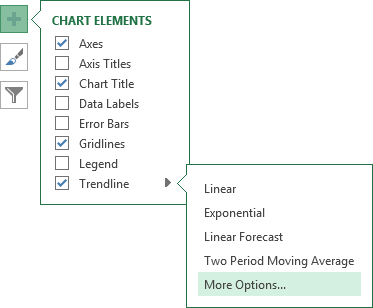You should see a panel open on the right of Excel, like the one in the next image.

For Excel 2007 and 2010 users, Click the Layout tab again. Then click the Trendline on the Analysis panel. From the Trendline menu this time, select More Trendline Options. You'll then see a dialogue box with options the same as the ones in the image below.The Trendline option we've chosen is Linear. Have a look at the bottom, and check the box next to Display Equation on chart.

When you check the box you should the following equation appear on your chart:

y = 564.88x + 13604

This is something called the Slope-Intercept Equation. If you remember you Math lessons from school, the equation is usually written like this (the "b" at the end may be a different letter, depending on where in the world you were taught Math):

y = mx + b

In this formula, the letter "m" is the slope (gradient) of the line, and the letter "b" is the first value on the y axis. The x is a value on the X-Axis. Once you have the slope of the line, a value for the X-Axis, and the starting point of the line, you can extend the line, and work out other values on it. This will be the letter "y" in the equation.

Excel has already worked out two values for us, the "m" and the "b". The "m" (the slope) is 564.88 and the "b" is an income value of 13604.

To work out the y values we just need an "x". The "x" for us will be those "Years since 2006" in our B column.

=564.88 * B10 + 13604

Press the enter key and you should find that Excel comes up with a value of 18123.04. This is the predicated income for the year 2014. Use Autofill for the cells B11 to B15. The rest of the predicted values will then be filled in: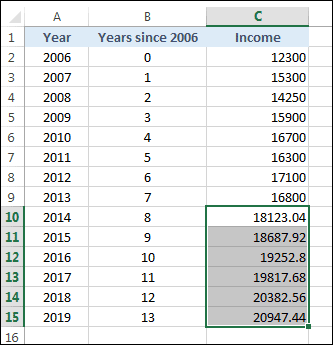So Excel is predicting we'll earn 18123.04 in 2014. By 2019, it's predicting we'll earn 20947.44.

In the next part of this tutorial, you'll see how to extend the Trendline so that these values are added to your chart.

<--Back to the Excel Contents Page